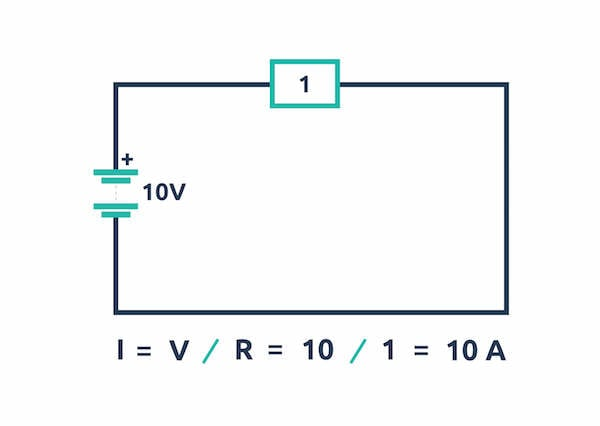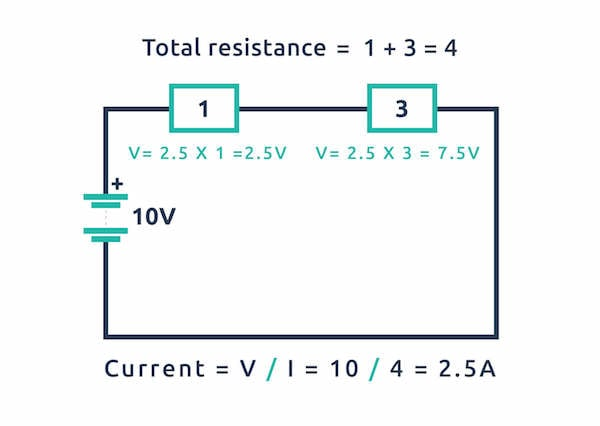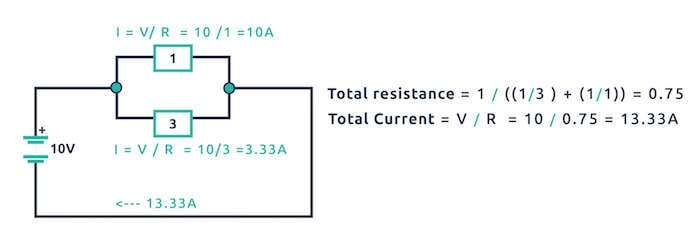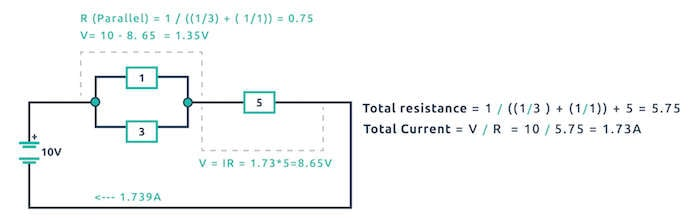Custom

# What is Ohm's Law? An introduction to Power SourcesSeptember 05, 2018 by Robin Mitchell
ShareGet introduced to voltage, current, and resistance and learn how they relate to one another in Ohm's Law.

For electronic systems to work they require power in some shape or form. Power can be found in many sources including wall adaptors (like those that charge phones), batteries, solar panels, and even wind turbines. But which power source you need to use depends on your project. If, for example, you are building a project that stands still on a table or wall should probably be powered by a mains adaptor (cheaper and more convenient than batteries), but if your project is to be used outside then a solar panel may be a good option. If your project intends to move around (such as a remote- controlled car), then batteries may be better than a solar panel as batteries contain a lot of power while not needing direct sunlight to work).

### Voltage, Current, and Resistance

Voltage, current, and resistance are arguably the three fundamentals to electronics and chances are you will need to deal with them at some point! But what are these things and how do they relate to each other?

When an electrical circuit is completed (i.e., there is a conductive path between the terminals of a power source such as a battery or mains connection), electrons move from the negative terminal to the positive terminal through the conductive path. This flow of electrons is called current but why did this current flow in the first place? For electrons to move they need some kind of force to push them along and this is what voltage does!

A voltage supply provides something called a potential difference, which can be thought of as a pulling force for electrons. If two battery terminals are connected through a wire, the positive terminal pulls the electrons to it and the negative terminal pushes them away. So, what stops all the electrons from one side of a battery going to the other side instantly when a conductive path is made between the terminals? This is where resistance comes in!

Resistance can be thought of as the ability of a material to resist the flow of electrons and a higher resistance results in the electrons finding it more difficult to pass through. To understand this better, an analogy will be used.

Imagine current as people moving through a corridor and a voltage supply as being people who are trying to push people through the corridor. If those â€œpushersâ€ (voltage source) want more people to get through the corridor (a higher current) then they need to push harder (higher voltage). But people can only go through the corridor so fast and if there is a narrow door in the middle that everyone needs to get through (a resistance), then the number of people moving through will be lower. Therefore, the rate at which people can move through the corridor is dependent on how hard the pushers push and how narrow the doorway is.

Going back to voltage, current, and resistance, the three are related in a very famous equation known as Ohm's Law:

V=IR

However, this equation is misleading as it implies that voltage is the result of current and resistance which is not true. Current is the result of voltage and resistance and the equation should be written as:

I=V/R

Voltage, current, and resistance all have their own units of measurements, names, and symbols:

• Voltage is measured in volts (V)
• Current is measured in amps (A)
• Resistance is measured in ohms (â„¦)

### Using Ohm's Law

Using V=IR must be done with caution as some get confused which voltage to use. What this equation essentially tells us is that the voltage across a resistor will be equal to the resistance multiplied by the current flowing through it. The best way to see this in action is to see some simple examples.

The first example shows a resistor of 1â„¦ connected to a 10V battery and the resulting current through the resistor is 10A.The second example shows two resistors in series and the voltage drops across each resistor.The third example shows two resistors in parallel, the voltage drops across them, and the current through each resistor.The fourth example shows two resistors in parallel all in series with a resistor. Notice anything interesting about the voltages?If you have not noticed by now all the voltages in a complete loop (from + terminal to â€“ terminal), add up to the supply voltage and this is called Kirchoffâ€™s Voltage Law! This law can be handy when trying to determine the voltage across a component if you know the voltage drop across all the other components in series. But voltages in parallel are always the same which can be handy to calculate currents across components in parallel.

A quick recap:

• Voltages in series add up
• Voltages in parallel are the same

### AuthorRobin Mitchell

Graduated from the University Of Warwick in Electronics with a BEng 2:1 and currently runs MitchElectronics.